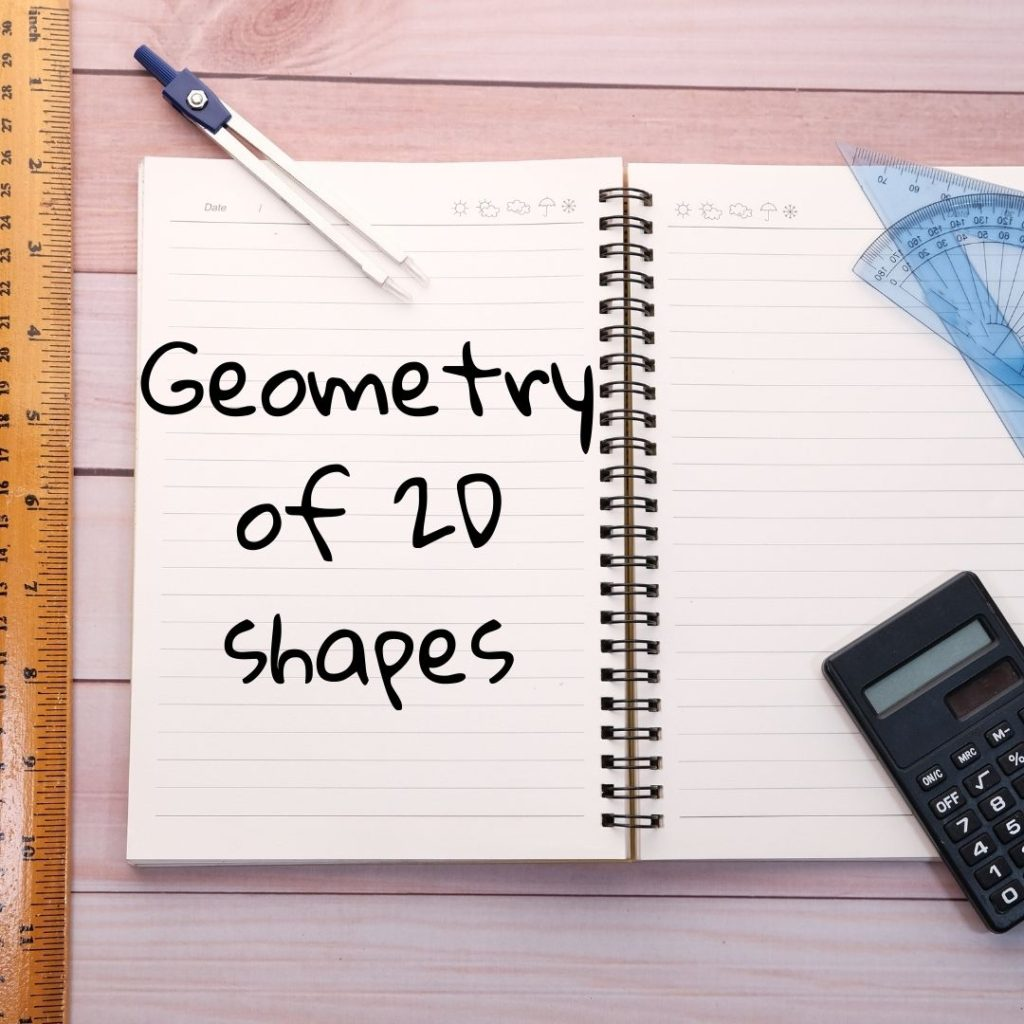# Geometry of 2d shapes grade 9

## Geometry of 2d shapes grade 9

In Grade 9 Geometry, you will learn about 2D shapes and their properties. Here are some of the key topics you can expect to cover:

1. Lines and angles:
• Types of lines: straight, curved, horizontal, vertical, diagonal, parallel and perpendicular lines
• Types of angles: acute, obtuse, right, straight, reflex and complementary angles
1. Polygons:
• Definition and properties of polygons
• Types of polygons: triangles, quadrilaterals, pentagons, hexagons, etc.
• Properties of each type of polygon: number of sides, angles, diagonals, perimeter and area
1. Circles:
• Definition and properties of circles
• Parts of a circle: radius, diameter, chord, tangent, sector and arc
• Circumference and area of a circle
1. Transformations:
• Translation: moving a shape from one position to another without changing its size or shape
• Reflection: flipping a shape over a line of reflection
• Rotation: turning a shape around a fixed point
1. Congruent and Similar shapes:
• Definition and properties of congruent and similar shapes
• Identifying congruent and similar shapes
• Proving that shapes are congruent or similar using mathematical reasoning

#### Geometry of 2D shapesThis grade 9 maths worksheet looks at the term 2 section of Geometry of 2D shapes.

It includes theory questions about triangles (scalene, isosceles, equilateral, and right-angled) and quadrilaterals (trapezium, parallelogram, rectangle, square, rhombus and kite).